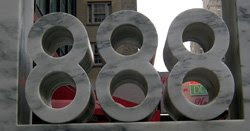## Friday, June 19, 2009

### 888

888 = 23 x 3 x 37.

888 is the only number whose cube (700227072) consists of three digits each occurring three times.

888 and the following 18 integers are all composite numbers.

888 is 4040 in base 6.

888 is the sum of eight consecutive primes: 97 + 101 + 103 + 107 + 109 + 113 + 127 + 131 = 888.888 is the number to key in for the letter “T” on a telephone keypad when text messaging.

Source: Number Gossip